# Test: Basic Electronics- 1

## 20 Questions MCQ Test Mock Test Series for SSC JE Electrical Engineering | Test: Basic Electronics- 1

Description
Attempt Test: Basic Electronics- 1 | 20 questions in 12 minutes | Mock test for SSC preparation | Free important questions MCQ to study Mock Test Series for SSC JE Electrical Engineering for SSC Exam | Download free PDF with solutions
QUESTION: 1

### Which of the following is a unipolar diode?

Solution:

In a unipolar device current is due to only one type of carriers (i.e. electrons or holes), while in bipolar device current is due to both holes and electrons.

Schottky diode or hot-carrier diode is a junction of n-type semiconductor and metal, in it electrons are sole carriers.

QUESTION: 2

### The reverse bias characteristics of semiconductor of a semiconductor diode is shown in

Solution:

A diode in forward-bias conducts current just like normal conductor, while in reverse-bias prohibits current and only very small amount of current flows, called reverse saturation current.

After certain reverse voltage called reverse breakdown voltage the diode breaks down and conducts huge current.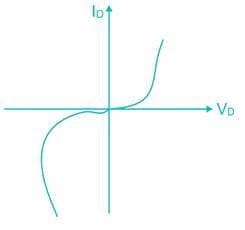QUESTION: 3

### The phenomenon of thermal run away is associated with

Solution:

Thermal runaway is a situation in which increase in temperature changes the conditions in a way that further causes temperature to increase.

In BJTs the collector current dissipates lot of heat, this increase in temperature causes increased flow of minority carriers from collector to base, further increasing the collector current. This increase in collector current further raise temperature, thus thermal runaway occurs ending in burnout of BJT.

QUESTION: 4

What are the ON/OFF terminals of a transistor when it is operated as a switch?

Solution:

Transistor switches can be used to switch low DC voltages ON or OFF by operating it in saturation or cut-off mode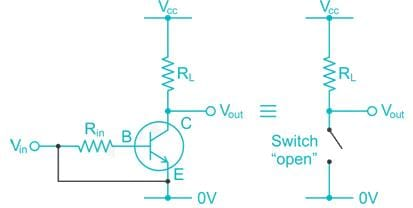The CE terminals act as ON/OFF terminals as shown in figure above.

QUESTION: 5

The LED emits light of a particular colour because

Solution:

LEDs are fabricated using direct bandgap semiconductors.In which, when electron transfers from conductor band to valence band and recombines with the holes the energy difference between the both bandsget converted in to light with frequency ‘ν’, it is related with energy as,

E = hν, where h is Planck’s constant.

QUESTION: 6

UJT are used in

Solution:

Uni Junction Transistor is a three terminal semiconductor device formed by single P-N junction. It functions as an ON-OFF switch and has unidirectional conductivity and negative resistance characteristics.

It is used to trigger SCR devices and used in relaxation oscillator.

QUESTION: 7

In JFET, the application of reverse bias to the gate terminals will cause the depletion layers to

Solution:

In JFET Gate and channel are opposite type of semiconductors with depletion layers in between them.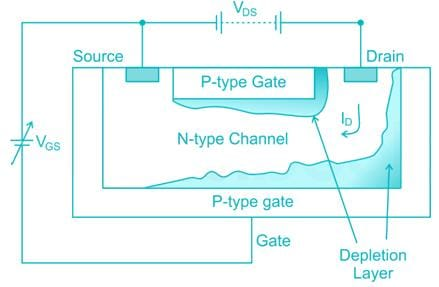If reverse voltage is applied to Gate terminal the depletion region increases and channel width is reduced, this effect is called pinch-off effect, limiting the drain current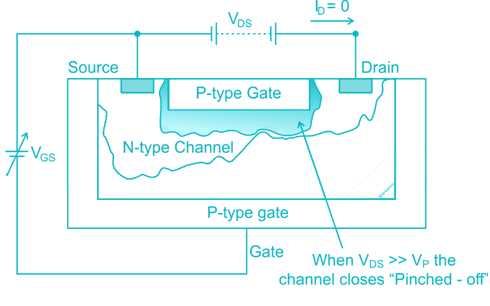QUESTION: 8

Two p-n junction diodes are connected back to back to make a transistor. Which one of the following is correct?

Solution:

A transistor operation needs very small base width to allow the minority carriers, drifted from emitter to diffuse into collector.

By connecting two PN diodes back to back the transistor will have wide base causing the minority carriers from emitter to recombine in base region before they diffuse into collector. This reduces collector current or cut off entirely.

QUESTION: 9

The gain of a CE amplifier is highest at

Solution:

A common emitter amplifier acts as a bandpass amplifier due to reduced gain at low and high frequencies. This is due to source, emitter and parasitic capacitances.

QUESTION: 10

In an electronic circuit transistor is used for switching ON and OFF a relay when the transistor switches OFF the relay a higher voltage appears across the transistor. How can a transistor be protected from this voltage?

Solution:

In transistor relay circuit when relay is in OFF state, high reverse voltage is developed across relay terminals, this can damage the transistor. Hence a diode is connected parallel to the relay, this clamps the voltage across the relay and helps dissipate the energy in diode.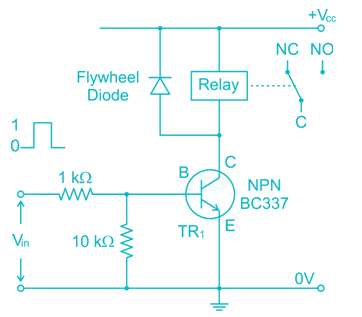QUESTION: 11

Which of the following is a bipolar device?

Solution:

Gunn diode, FET and UJT are unipolar devices in which current is due to only one type of carrier. While tunnel diode is bipolar device in which both electrons and holes contribute to current.

QUESTION: 12

Transistor biasing is done to keep _______ in the circuit.

Solution:

Biasing of a transistor is done to keep the stable DC condition like VBE, IC needed for specific action of transistor, like amplification, switch, etc.

QUESTION: 13

In an amplifier the maximum power transfer to the load resistance should be

Solution:

In an amplifier the maximum power is transferred to the load when the load resistance is equal to output impedance of amplifier

QUESTION: 14

What is the main source of distortion in a push-pull amplifier?

Solution:

In push-pull amplifiers each transistor operates only for one half cycle of AC voltage, in it even harmonics are minimised, so distortion is mainly due to odd harmonics.

Hence of the given options third harmonics needs to be eliminated

QUESTION: 15

The bonding forces in compound semiconductors, such as GaAs, arise form

Solution:

In semiconductors like GaAs the elements Ga and As are in covalent bond. Arsenic has five valence electrons while gallium has three valence electrons. Therefore, in GaAs crystal Arsenic contribute one electron to Gallium and it is shared between both atoms in a covalent bond

QUESTION: 16

ICBO in a transistor can be reduced by reducing:

Solution:

ICBO is the reverse leakage current between collector and base while emitter is open it is given by ICBO=(1−α)IC−αIB. It is the reverse current in base collector junction, which is dependent on temperature at the junction and is reduced with decreasing current.

QUESTION: 17

Zener diodes are used as:

Solution:

Zener diodes in reverse bias starts conduct current at a certain reverse voltage known as Zener voltage. Above the Zener voltage the diode maintains constant voltage across its terminals for varying values of current, hence Zener diodes are used as voltage regulators.

QUESTION: 18

The Trans conductance of a JFET is computed at constant VDS by:

Solution:

In a JFET transconductance is defined as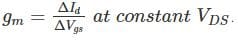Hence it is computed at constant VDS by ratio of change in lto change of Vgs.

QUESTION: 19

The intersection of the D.C. load line with the given base current curve is the

Solution:

The intersection of DC load line with the parameter graph of a semiconductor device (for example base current vs VCE in transistor) gives operating point of the said device

QUESTION: 20

Which among the following will possess a higher bandwidth, if two transistors are provided with unity gain frequency?

Solution:

In transistor the Gain Bandwidth product is equal to unity, since gain is proportional to DC current gain hfe the transistor with lower hfe will have higher bandwidth.Use Code STAYHOME200 and get INR 200 additional OFF Use Coupon Code